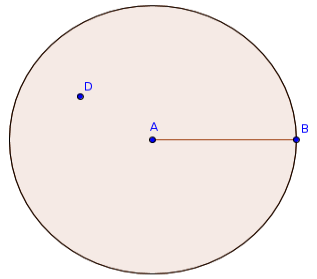Welcome guest
You're not logged in.
277 users online, thereof 0 logged in

## Definition: 1.15: Circle, Circumference, Radius

### (Definition 15 from Book 1 of Euclid’s “Elements”)

A circle is a plane figure contained by a single line [which is called a circumference], (such that) all of the straight lines radiating towards [the circumference] from one point amongst those lying inside the figure are congruent to one another.

### Modern Formulation

Let $$A,B$$ be two different points in a plane $$\mathcal P$$, and let $$d(A,B)$$ denote their Euclidean distance (i.e. the length of the segment $$\overline{AB}$$). A circle is a plane figure consisting of points of the plane $$D$$, which have a smaller or equal distance from $$A$$, formally

$\text{Circle}:=\{D\in\mathcal P:~d(A,D)\le d(A,B)\}.$

Any segment $$\overline{A,D}$$ with maximum possible distance $$d(A,D)=d(A,B)$$ is called the radius of the circle.

The circumference of the circle is the boundary of the circle, i.e. all points $$D$$ in the plane, which have the maximum possible distance:

$\text{Circlumference}:=\{D\in\mathcal P:~d(A,D) = d(A,B)\}.$

### Example

A circle with a radius $$\overline{AB}$$. In the figure, one of the infinitely many points $$D$$ with $$d(A,D)\le d(A,B)$$ is marked.### CC BY-SA 4.0

 Callahan, Daniel: “Euclid’s ‘Elements’ Redux”, http://starrhorse.com/euclid/, 2014